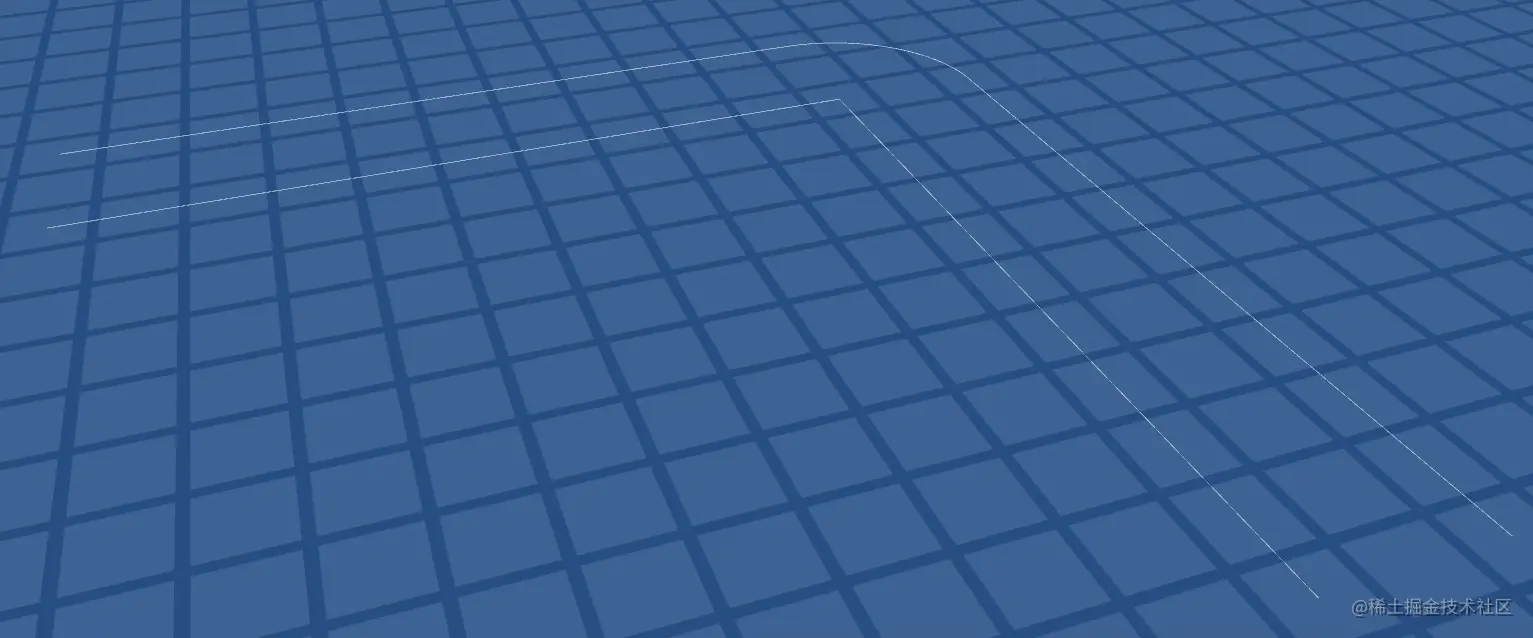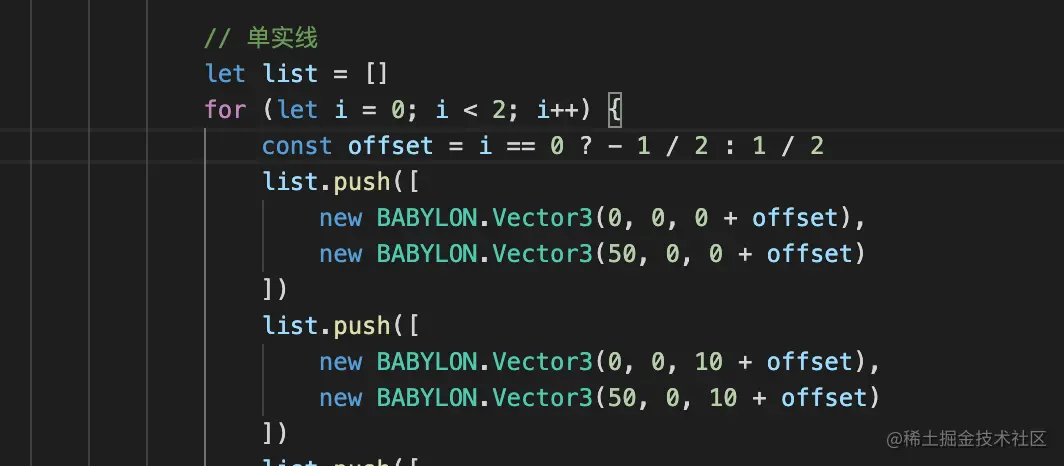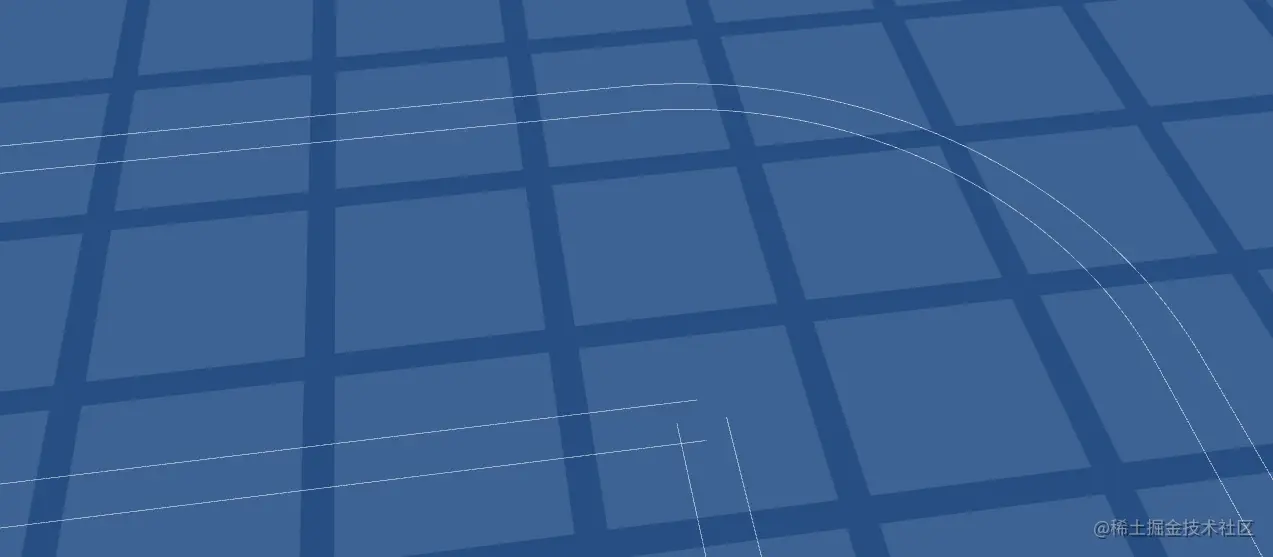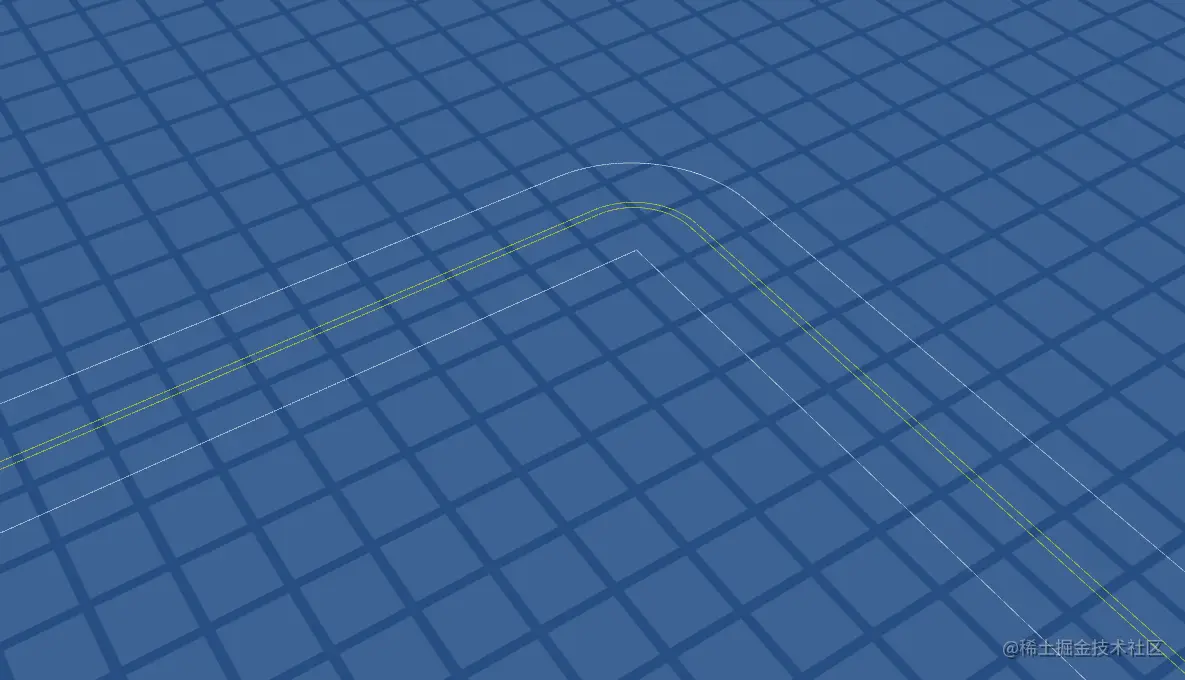# [babylonjs] babylonjs practice (XII) -- drawing lane lines

## background

The previous section talked about independent line drawing . This chapter is combined with an example. Let's draw a .

Example ： Draw lane line

## Need to be disassembled

We got the road data , It is essentially a line segment , Just draw it directly .

But we should not distinguish between different line types ..

## Text

### Single solid line

Took five lines of data .

``````          //  Single solid line
const line11 = [
new BABYLON.Vector3(0, 0, 0),
new BABYLON.Vector3(50, 0, 0)
]
const line12 = [
new BABYLON.Vector3(0, 0, 10),
new BABYLON.Vector3(50, 0, 10)
]
const line21 = [
new BABYLON.Vector3(0, 0, 10),
new BABYLON.Vector3(0, 0, 60)
]
const line22 = [
new BABYLON.Vector3(-10, 0, 10),
new BABYLON.Vector3(-10, 0, 60)
]
var line33 = [];
for(let i=180; i<=270; i++){
line33.push(new BABYLON.Vector3(10* Math.sin(i/180*Math.PI), 0, 10 + 10 * Math.cos(i/180*Math.PI)));
}

const singleSolidLineOptions = {
lines: [line11, line12, line21, line22, line33],
useVertexAlpha: false
}
BABYLON.MeshBuilder.CreateLineSystem('', singleSolidLineOptions, scene)

Copy code ``````### Line width

This problem can't be solved for the time being Did not find width Configuration item for . forum.babylonjs.com/t/setting-l…

Make an attempt Then draw two lines to deal with . But the linewidth 1px Actually, that's not the case when you show it .no way .

Another attempt is to use a rectangle .

If you use a rectangular entity, you will not be able to see clearly if it is far away , Rendering with lines won't have this problem . Remember when you drew the vehicle before , It wasn't drawn in wireframe at first , Looking down from a very high sky, the car looks very blurred .

Temporarily abandon .

### Double yellow line

``````     const computeOffsetPoint = (targetPoint, referencePoint, changeSide = false) => {
const offsetDirection = referencePoint.subtract(targetPoint).cross(new BABYLON.Vector3(0, 1, 0)).normalize()
const offsetDimension = 0.3

if (changeSide) {
offsetDirection.scaleInPlace(-1.0)
}

return offsetPoint
}

//  Double yellow line
const line51 = [
computeOffsetPoint(new BABYLON.Vector3(0, 0, 5),new BABYLON.Vector3(50, 0, 5)),
computeOffsetPoint(new BABYLON.Vector3(50, 0, 5),new BABYLON.Vector3(100, 0, 5)),
]
const line52 = [
computeOffsetPoint(new BABYLON.Vector3(-5, 0, 10),new BABYLON.Vector3(-5, 0, 60)),
computeOffsetPoint(new BABYLON.Vector3(-5, 0, 60),new BABYLON.Vector3(-5, 0, 110)),
]
var line53 = [];
for (let i = 180; i <= 270; i++) {
line53.push(new BABYLON.Vector3(5 * Math.sin(i / 180 * Math.PI), 0, 10 + 5 * Math.cos(i / 180 * Math.PI)));
}
var line54 = []
for(let i = 0; i<line53.length-1; i++){
line54.push(computeOffsetPoint(line53[i], line53[i+1]))
}

const line511 = [
computeOffsetPoint(new BABYLON.Vector3(0, 0, 5),new BABYLON.Vector3(50, 0, 5), true),
computeOffsetPoint(new BABYLON.Vector3(50, 0, 5),new BABYLON.Vector3(100, 0, 5), true),
]
const line521 = [
computeOffsetPoint(new BABYLON.Vector3(-5, 0, 10),new BABYLON.Vector3(-5, 0, 60), true),
computeOffsetPoint(new BABYLON.Vector3(-5, 0, 60),new BABYLON.Vector3(-5, 0, 110), true),
]
var line541 = []
for(let i = 0; i<line53.length-1; i++){
line541.push(computeOffsetPoint(line53[i], line53[i+1], true))
}

const options22 = {
lines: [line51, line52, line54, line511, line521, line541],
useVertexAlpha: false
}
var line = BABYLON.MeshBuilder.CreateLineSystem('', options22, scene)
line.color = new BABYLON.Color3(1,1, 0)

Copy code ``````

The effect is as followsOne more thing to say again . involve computeOffsetPoint This method .

## Real map drawing

That's the difference between data sources .

The data of the map is directly from ue Derived from , And turn it into json Format .

The format of data sources in each project is different , When processing, the corresponding processing is OK .

``````	"roadLine": [
{
"BoundaryPoints": [
{
"x": 349.93106079101563,
"y": -202.75816345214844,
"LineType": "solid",
"LineColor": "white"
},

...
Copy code ``````

Such a sub format , A road consists of BoundaryPoints Data composition of .

Each element is a road point , Indicates the coordinates , And the style and color of the line out of this point .

https://cdmana.com/2021/09/20210909122358669b.html

Scroll to Top